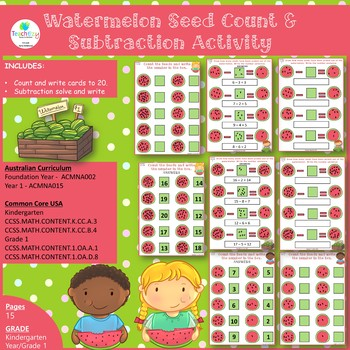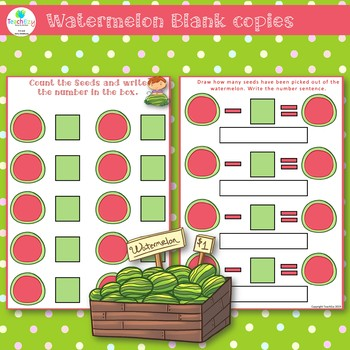# Watermelon Seed Count and Subtraction Activity Kindergarten and Year or Grade 1Subject
Resource Type
File Type

PDF

(1 MB|15 pages)
Product Rating
Standards
• Product Description
• StandardsNEW

Watermelon seed count and subtraction activity suitable for kindergarten and Year or Grade 1. In the first activity, students count the watermelon seeds and write the number of seeds. In the subtraction activity, students determine the unknown whole number in the number sentence and then write the number sentence.

Pages: Includes 1 teacher instructions page, 6 pages with 6 pages of answers and 2 blank pages for creating your own.

Format: PDF

• Foundation Year ACMNA002
• Year 1 - ACMNA015

Links to Common Core USA -

• Kindergarten CCSS.MATH.CONTENT.K.CC.A.3 and CCSS.MATH.CONTENT.K.CC.B.4
• Grade 1 CCSS.MATH.CONTENT.1.OA.A.1 and CCSS.MATH.CONTENT.1.OA.D.8
Determine the unknown whole number in an addition or subtraction equation relating three whole numbers. For example, determine the unknown number that makes the equation true in each of the equations 8 + ? = 11, 5 = ▯ - 3, 6 + 6 = ▯.
Use addition and subtraction within 20 to solve word problems involving situations of adding to, taking from, putting together, taking apart, and comparing, with unknowns in all positions, e.g., by using objects, drawings, and equations with a symbol for the unknown number to represent the problem.
Understand the relationship between numbers and quantities; connect counting to cardinality.
Write numbers from 0 to 20. Represent a number of objects with a written numeral 0-20 (with 0 representing a count of no objects).
Total Pages
15 pages
N/A
Teaching Duration
N/A
Report this Resource to TpT
Reported resources will be reviewed by our team. Report this resource to let us know if this resource violates TpT’s content guidelines.